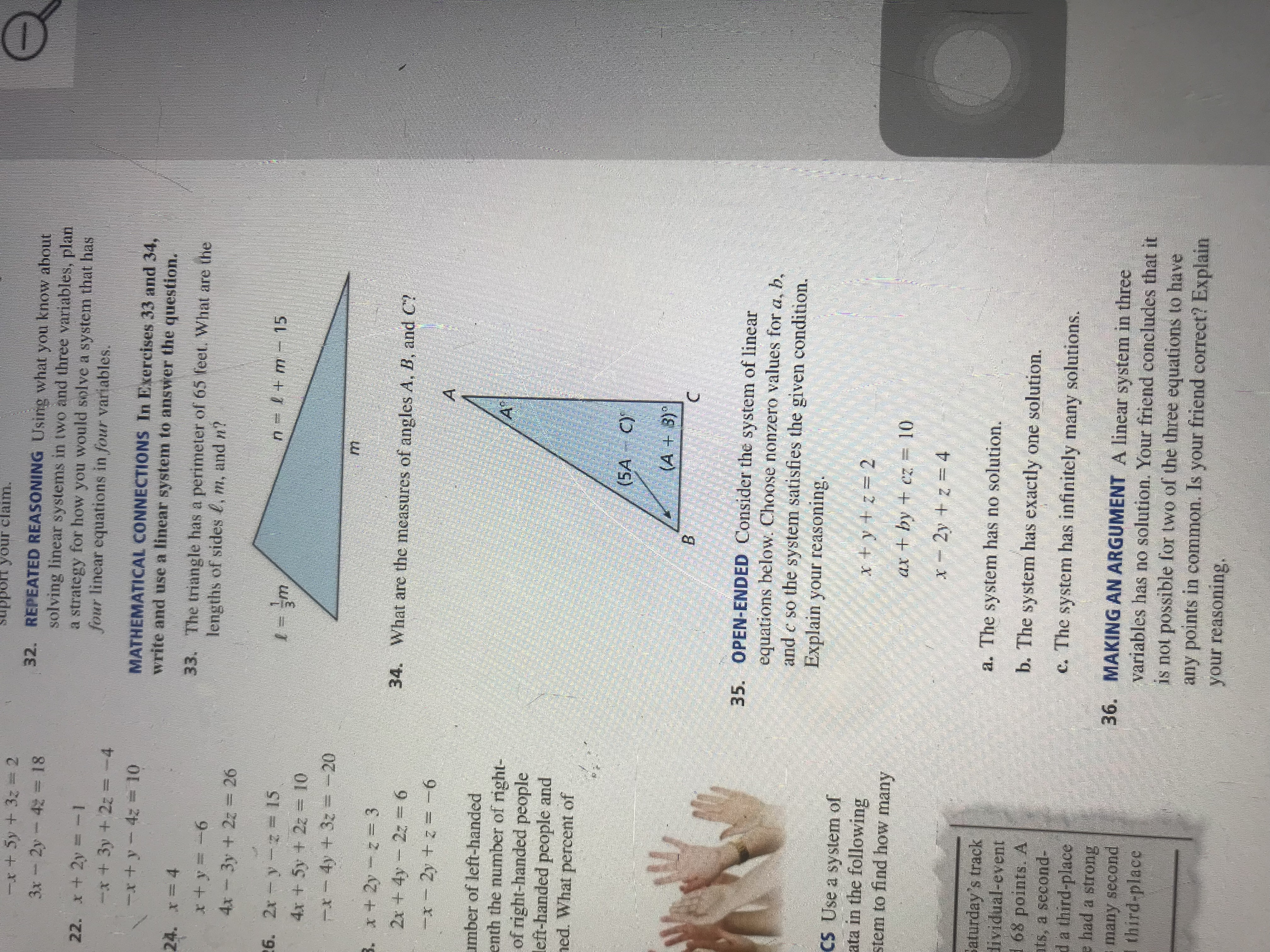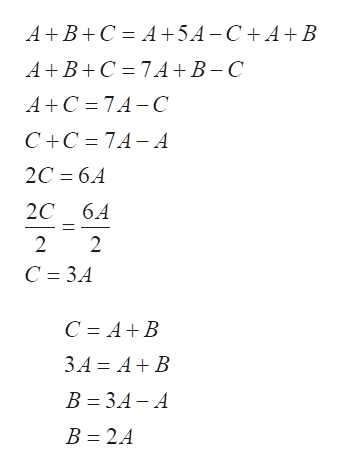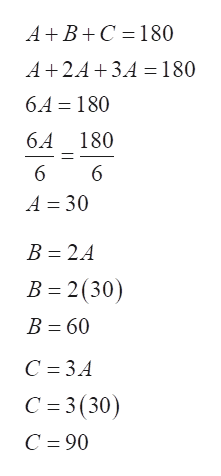# oIt your claim.-t + 5y +3z232. REPEATED REASONING Using what you know aboutsolving linear systems in two and three variables, plana strategy for how you would solve a system that hasfour linear equations in four variables.3x 2y 41822. x 2yx +3y +2z=-4+y-4 101MATHEMATICAL CONNECTIONS In Exercises 33 and 34,write and use a linear system to answer the question.24. x=433. The triangle has a perimeter of 65 feet. What are thelengths of sides 4, m, and n?4x 3y + 2z26n +m 15- m26. 2x y= 154x + 5y +2z10t-4y +3z= -20B. x 2y z32x + 4y 2z 634. What are the measures of angles A, B, and Cx2y +z -6Aumber of left-handedenth the number of right-of right-handed peopleleft-handed people andned. What percent ofA(5A C)(A+3)BC35. OPEN-ENDED Consider the system of linearequations below. Choose nonzero values for a, band c so the system satisfies the given condition.Explain your reasoning.CS Use a system ofata in the followingstem to find how manyx ty+z= 2+ by +cz 102y z 4Saturday's tracklividual-event168 points. Ats, a second-da third-placee had a stronghas no solution.a. The systemb. The system has exactlyone solution.,C. The system has infinitely many solutions.36. MAKING AN ARGUMENT A linear system in threevariables has no solution. Your friend concludes that itis not possible for two of the three equations to haveany points in common. Is your friend correct? Explainyour reasoningmany secondthird-place

Question
33 viewshelp_outlineImage TranscriptioncloseoIt your claim. -t + 5y +3z2 32. REPEATED REASONING Using what you know about solving linear systems in two and three variables, plan a strategy for how you would solve a system that has four linear equations in four variables. 3x 2y 418 22. x 2y x +3y +2z=-4 +y-4 10 1 MATHEMATICAL CONNECTIONS In Exercises 33 and 34, write and use a linear system to answer the question. 24. x =4 33. The triangle has a perimeter of 65 feet. What are the lengths of sides 4, m, and n? 4x 3y + 2z 26 n +m 15 - m 26. 2x y = 15 4x + 5y +2z 10 t-4y +3z= -20 B. x 2y z3 2x + 4y 2z 6 34. What are the measures of angles A, B, and C x2y +z -6 A umber of left-handed enth the number of right- of right-handed people left-handed people and ned. What percent of A (5A C) (A+3) B C 35. OPEN-ENDED Consider the system of linear equations below. Choose nonzero values for a, b and c so the system satisfies the given condition. Explain your reasoning. CS Use a system of ata in the following stem to find how many x ty+z= 2 + by +cz 10 2y z 4 Saturday's track lividual-event 168 points. A ts, a second- da third-place e had a strong has no solution. a. The system b. The system has exactly one solution., C. The system has infinitely many solutions. 36. MAKING AN ARGUMENT A linear system in three variables has no solution. Your friend concludes that it is not possible for two of the three equations to have any points in common. Is your friend correct? Explain your reasoning many second third-place fullscreen
check_circle

Step 1

Given triangle is:

From the given triangle,

A = A, B = 5AC, C = A + B

Step 2

Here, the sum of angles is given by:

Plugging the value of C in the angle C,help_outlineImage TranscriptioncloseA B C A54-C+A+B A BC 7 A +B-C AC 7A-C C+C 7A- A 2C 6.4 2C 6A 2 2 C 3.4 C A B 3A AB B 3A- A B 2.A fullscreen
Step 3

The sum of angles in a triangle is 180 degrees.

Hence,

Plugging the value of A, to obtain the values of B and C:

Hence, the...help_outlineImage TranscriptioncloseABC 180 A 2.A 3A= 180 6.A 180 6.A 180 6 6 A 30 B 2.A B 2(30) B 60 C 34 C 3(30) C 90 fullscreen

### Want to see the full answer?

See Solution

#### Want to see this answer and more?

Solutions are written by subject experts who are available 24/7. Questions are typically answered within 1 hour.*

See Solution
*Response times may vary by subject and question.
Tagged in

### Algebra